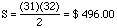Date: Sun, 08 Nov 1998 21:16:15 -0700
Subject: Depositing money. . .

It has been years since I was in school and I can't remember if there is a formula for the following problem:

If you deposit \$1.00 on the first day of the month, \$2.00 on the second day, \$3.00 on the third day . . \$31.00 on the last day of the month, how much do you have in the bank?

Now will this formula also work if it was, \$0.25 (then day two you would deposit 2x \$0.25 or \$0.50, day three you would deposit 3x \$0.25, \$0.75. . . ). Will it work with any denomination??

Thank you for your time. I promise I'll write this one down for future reference. . .

Hi Gerry

The way the problem is stated you are not collecting any interest on your money. Thus what you want is the sum

S = 1 + 2 + 3 + ... + 31.

I won't tell you a formula as it's too easy to forget but I will show you how to do the sum.

Write the sum twice, the second time in reverse order.

 S = 1 + 2 + 3 + ... 29 30 31 S = 31 + 30 + 29 + ... 3 2 1

 2S = 32 + 32 + 32 + ... 32 32 32
So

2S = 31(32)
and thusYour second problem is handled in the same way, write the sum twice, the second time in reverse order and then add down.

Cheers
Penny

Go to Math Central